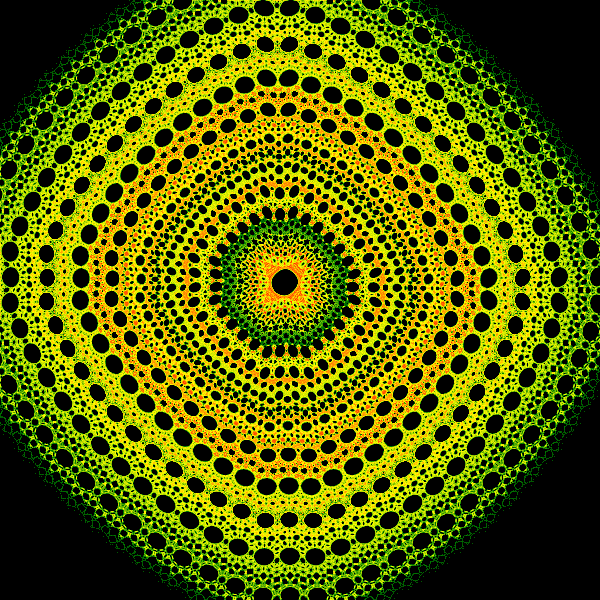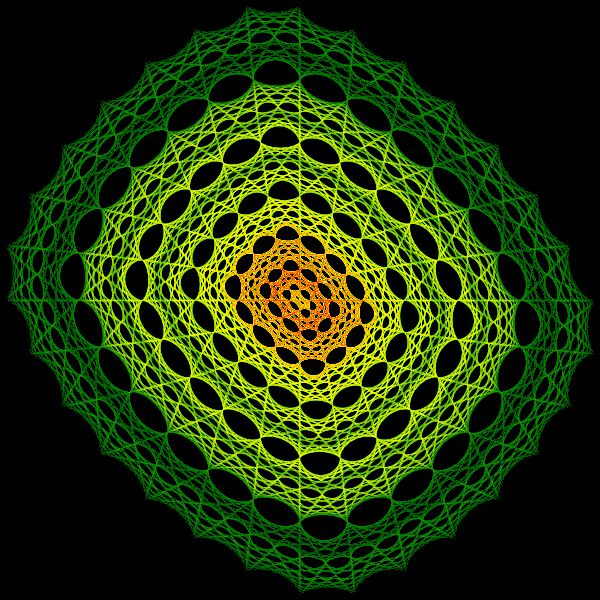# Hopalong fractal in generativepy

Martin McBride
2021-12-17

The Hopalong fractal is a strange attractor that looks quite different to the fractals we have seen before (such as Tinkerbell and King's Dream). It fills the plane, leaving an interesting pattern of holes.

The Hopalong fractal was discovered by Dr Barry Martin.

The fractal is not a true attractor, because the pattern obtained depends on the parameters A, B, C, and also the initial values of x and y. In a true attractor, you would always get the same pattern regardless of the initial x and y. But that is something of a technicality. It works in the same way as an attractor, and it produces fractal patterns. Just be aware that if you alter the initial values of x and y the result might change.

It is worth reading the coloured Tinkerbell fractal article before tackling this fractal.

## Hopalong equations

The fractal equations for Hopalong are:

xnext = y-math.sqrt(abs(B*x-C))*sign(x)
ynext = A-x


The sign function is a function that returns the following value:

• +1 if x is greater than 0.
• -1 if x is less than 0.
• 0 if x is equal 0.

## The code

Here is the full code for the Hopalong fractal:

from generativepy.bitmap import Scaler
from generativepy.nparray import make_nparray_data, save_nparray, load_nparray, make_npcolormap, apply_npcolormap, save_nparray_image
from generativepy.color import Color
from generativepy.utils import temp_file
from generativepy.analytics import print_stats, print_histogram
import numpy as np
import math

MAX_COUNT = 10000000
A = -55
B = -1
C = 42

def sign(x):
if x > 0:
return 1
if x < 0:
return -1
return 0

def colorise(counts):
counts = np.reshape(counts, (counts.shape, counts.shape))
power_counts = np.power(counts, 0.25)
maxcount = np.max(power_counts)
normalised_counts = (power_counts * 1023 / max(maxcount, 1)).astype(np.uint32)

colormap = make_npcolormap(1024, [Color('black'), Color('green'), Color('yellow'), Color('red')])

outarray = np.zeros((counts.shape, counts.shape, 3), dtype=np.uint8)
apply_npcolormap(outarray, normalised_counts, colormap)
return outarray

def paint(image, pixel_width, pixel_height, frame_no, frame_count):
scaler = Scaler(pixel_width, pixel_height, width=1000, startx=-500, starty=-500)

x = -1
y = 0
for i in range(MAX_COUNT):
x, y = y-math.sqrt(abs(B*x-C))*sign(x), A-x
px, py = scaler.user_to_device(x, y)
if 0 <= px < pixel_width and 0 <= py < pixel_height:
image[py, px] += 1

filename = temp_file('hopalong.dat')

data = make_nparray_data(paint, 600, 600, channels=1)
save_nparray(filename, data)

frame = colorise(data)

save_nparray_image('hopalong.png', frame)


This code is available on github in blog/fractals/hopalong.py.

Here is the image it createsThis code is similar to the Tinkerbell implementation, the main changes are the formula used and the Scaler range.

Unlike Tinkerbell, which covers a very well defined area, the Hopalong fractal covers a very large area but is extremely faint around the edges. A more appealing image is obtained by cropping the area to the central section and ignoring the stray outer points. To do this, we must ignore any pixels that are outside the range:

        if 0 <= px < pixel_width and 0 <= py < pixel_height:
image[py, px] = 0


This is the same code we use in the Henon fractal.

## Variants

We can create variants of the Hopalong by changing the parameters A, B, and C. For any set of parameters, the shape might also be affected by the choice of initial x and y values.

That provides a lot of options, but not all of them will give pleasing results. It is a matter of trial and error. If you do find a set of values that look good, it is worth further experimenting to fine-tune the values - varying them slightly to see if the result look even better.

Here is a variant that uses the following parameters:

A = -55
B = -1
C = 42
x = -1 # Initial value
y = 0 # Initial value


Here is the result: